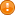Scilab Home page | Wiki | Bug tracker | Forge | Mailing list archives | ATOMS | File exchange
Change language to: English - Français - Português - Русский

See the recommended documentation of this function

Scilabヘルプ >> Elementary Functions > matrixmanipulation > permute

# permute

permutes the dimensions of an array

### Syntax

`y = permute(x, dims)`

### Arguments

x

an array with any number of dimensions (0, 1, 2, or any number n>2), of any data type: booleans, integers, decimal real or complex numbers, polynomials, rationals, array of structures, array of cells.

dims

a valid permutation of 1:n>=nmin where n is the number of dimensions of the desired array, at least as many as `nmin = ndims(x)`.

The dimension `#i` in `y` was the dimension `#dims(i)` in `x`.

y

an array with the contents of `x`, with identical but permuted sizes.

### Description

Permutes the dimensions of the array according to `dims`.Any trailing dimension of the result `y` that becomes a singleton (has a new size equal to 1, coming from a formerly non trailing singleton dimension) is automatically ignored (squeezed).`permute()` is mainly interesting when dealing with hypermatrices. To only transpose a matrix (permute its rows into columns and vice-versa), the `.'` or `'` operators should be prefered.

### Examples

```// Example 1: A matrix becomes an hypermatrix with one side of size==1
//            (here the number of rows)
x = [1 2 3; 4 5 6]
y = permute(x,[3 1 2])```
```--> x = [1 2 3; 4 5 6]
x  =
1.   2.   3.
4.   5.   6.

--> y = permute(x,[3 1 2])
y  =
(:,:,1)
1.   4.
(:,:,2)
2.   5.
(:,:,3)
3.   6.
```
```// Example 2: With encoded integers
x = int8(matrix(1:24, [3 4 2]))
y = permute(x, [2 3 1])```
```--> x = int8(matrix(1:24, [3 4 2]))
x  =
(:,:,1)
1  4  7  10
2  5  8  11
3  6  9  12
(:,:,2)
13  16  19  22
14  17  20  23
15  18  21  24

--> y = permute(x, [2 3 1])
y  =
(:,:,1)
1  13
4  16
7  19
10  22
(:,:,2)
2  14
5  17
8  20
11  23
(:,:,3)
3  15
6  18
9  21
12  24
```
```// Example 3:
x = matrix(1:8, [2 1 4])
y = permute(x, [3 1 2]);
// The dimension 1 was the 3rd: size = 4 = new number of rows
// The dimension 2 was the 1st: size = 2 = new number of columns
// The dimension 3 was the 2sd: size = 1 = new number of pages
y```

• pertrans — 第2対角項を基準として転置
• quote — (') 転置演算子, 文字列デリミタ
• ndims — 配列の次元の数
• squeeze — ハイパー行列のシングルトン次元を削除

### History

 バージョン 記述 6.0 Extension to rationals